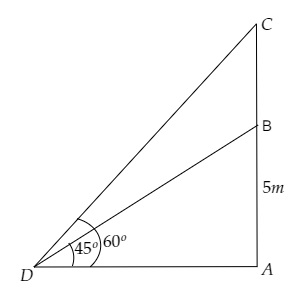# A flag-staff stands on the top of 5 m high tower. From a point on the ground, the angle of elevation of the top of the flag-staff is $60^{\circ}$ and from the same point, the angle of elevation of the top of the tower is $45^{\circ}$. Find the height of the flag-staff.

Given:

A flag-staff stands on the top of 5 m high tower.

From a point on the ground, the angle of elevation of the top of the flag-staff is $60^{\circ}$ and from the same point, the angle of elevation of the top of the tower is $45^{\circ}$.

To do:

We have to find the height of the flag-staff.

Solution:Let $AB$ be the tower and $BC$ be the length of the flag-staff.

Let point $D$ be the point of observation.

From the figure,

$\mathrm{AB}=5 \mathrm{~m}, \angle \mathrm{CDA}=60^{\circ}, \angle \mathrm{BDA}=45^{\circ}$

Let the height of the flag-staff be $\mathrm{BC}=h \mathrm{~m}$ and the distance between the tower and point $D$ be $\mathrm{AD}=x \mathrm{~m}$.

This implies,

$\mathrm{AC}=5+h \mathrm{~m}$

We know that,

$\tan \theta=\frac{\text { Opposite }}{\text { Adjacent }}$

$=\frac{\text { AB }}{DA}$

$\Rightarrow \tan 45^{\circ}=\frac{5}{x}$

$\Rightarrow 1=\frac{5}{x}$

$\Rightarrow x=5 \mathrm{~m}$...........(i)

Similarly,

$\tan \theta=\frac{\text { Opposite }}{\text { Adjacent }}$

$=\frac{\text { AC }}{DA}$

$\Rightarrow \tan 60^{\circ}=\frac{h+5}{x}$

$\Rightarrow \sqrt3=\frac{h+5}{5}$                 [From (i)]

$\Rightarrow 5\sqrt3=h+5 \mathrm{~m}$

$\Rightarrow h=5\sqrt3-5 \mathrm{~m}$

$\Rightarrow h=5(1.732-1) \mathrm{~m}$

$\Rightarrow h=5(0.732) \mathrm{~m}$

$\Rightarrow h=3.65 \mathrm{~m}$

Therefore, the height of the flag-staff is $3.65 \mathrm{~m}$.

Updated on: 10-Oct-2022

49 Views